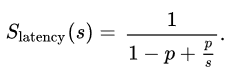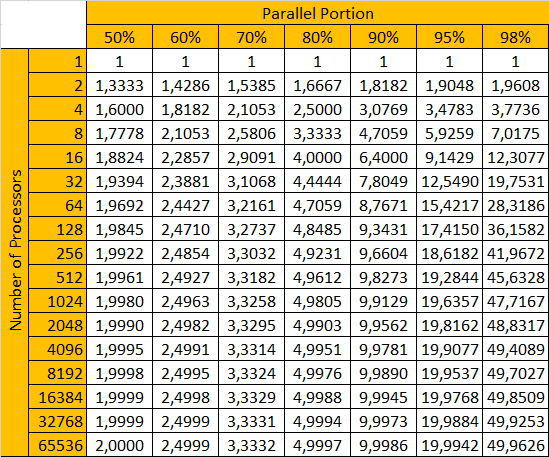When designing systems that need to scale you always need to remember that [tweet]more computing power does not necessarily mean better performance.[/tweet].

In this post, I will share a formula to compute the theoretical limit on the speedup possible, regarding latency to complete a task, for a given workload based on the proportional task that must be executed sequentially.

Let’s talk about the Amdahl’s Law.

## Hello, Amdahl’s Law

I think the best way to understand the Amdahl’s Law is with a simple example.

Assume that you need to perform data analysis for a 1 PB dataset, and this task takes 100 minutes to be completed. Also, assume that the dataset could be partitioned and processed in parallel without dependencies between the tasks. Sounds nice, right?

Now, assuming that the results of each of these parallel tasks need to be merged serially to produce results once the parallel tasks are complete. If the results processing takes ten minutes (10% of the runtime), then the overall latency for this job cannot be less than 10 minutes. In other words, no matter how much parallelism you have, you will need at least 10 minutes to complete the task.

## The Formula

The Amdahl’s law formula is:Where:

• p is a part that benefits of parallelism.
• s is the number of processors

Let’s do some simulations.As you can see, when 90% of the code can run in parallel, so, our speedup limit would be only ~10 times max. But it would be costly.

## Takeaways

Let’s finish this post with some takeaways.

• There is a theoretical limit on the speedup possible. Knowing how to calculate this limit is nice.
• The best way to improve theoretical limit on the speedup is reducing the non-parallel portion of the code
• Reducing the non-parallel portion of the code potentialy saves a lot of money.